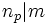Congruence condition on Sylow numbers

This article gives the statement, and possibly proof, of a constraint on numerical invariants that can be associated with a finite group

This article describes a congruence condition on an enumeration, or a count. It says that in a finite group and modulo prime number, the number of Sylow subgroups, i.e., the number of subgroups of prime power order whose index is relatively prime to the order satisfies a congruence condition.
View other congruence conditions | View divisor relations

Statement

Let$G$ be a finite group and$p$ a prime. Let$n_p$ be the$p$-Sylow number of$G$, i.e., the number of$p$-Sylow subgroups of$G$. Then:$n_p \equiv 1 \mod p$.

Since all the$p$-Sylow subgroups are conjugate,$n_p$ equals the index of any$p$-Sylow subgroup. Thus, this is equivalent to the following: if$P$ is a$p$-Sylow subgroup:$[G:N_G(P)] \equiv 1 \mod p$.

Related facts

Other parts of Sylow's theorem

• Sylow's theorem: The whole theorem.
• Sylow subgroups exist
• Sylow implies order-conjugate: Any two Sylow subgroups for the same prime are conjugate.
• Sylow implies order-dominating: Any$p$-subgroup is contained in some conjugate of any given$p$-Sylow subgroup.
• Divisibility condition on Sylow numbers: If the order of$G$ is$p^km$ where$m$ is relatively prime to$p$, then:$n_p | m$.

This fact is often used along with the congruence condition on Sylow numbers.

Generalizations

• Congruence condition on number of subgroups of given prime power order: Let$G$ be a finite group and$p^r$ be any prime power dividing the order of$G$. Then, the number of subgroups of order$p^r$ in$G$ is congruent to$1$ modulo$p$.
• Congruence condition on Sylow numbers in terms of maximal Sylow intersection: This states that if the intersection of two$p$-Sylow subgroups has index at least$p^k$, then$n_p$, the number$p$-Sylow subgroups, is congruent to$1$ modulo$p^k$.
• Congruence condition on number of Sylow subgroups containing a given subgroup of prime power order: This states that if$G$ is a finite group and$A$ is a$p$-subgroup of$G$, the number of$p$-Sylow subgroups of$G$ containing$A$ is congruent to$1$ modulo$p$.
• Congruence condition on factorization of Hall numbers for a finite solvable group.

Converse

A converse of sorts might be: whenever$a$ is a natural number such that$a \equiv 1 \mod p$, there exists a finite group$G$ such that$n_p = a$, i.e.,$a$ is the number of$p$-Sylow subgroups of$G$.

This is false. However, some partial converses are true:

Proof

This proof assumes that we already know that there exist$p$-Sylow subgroups of$G$.